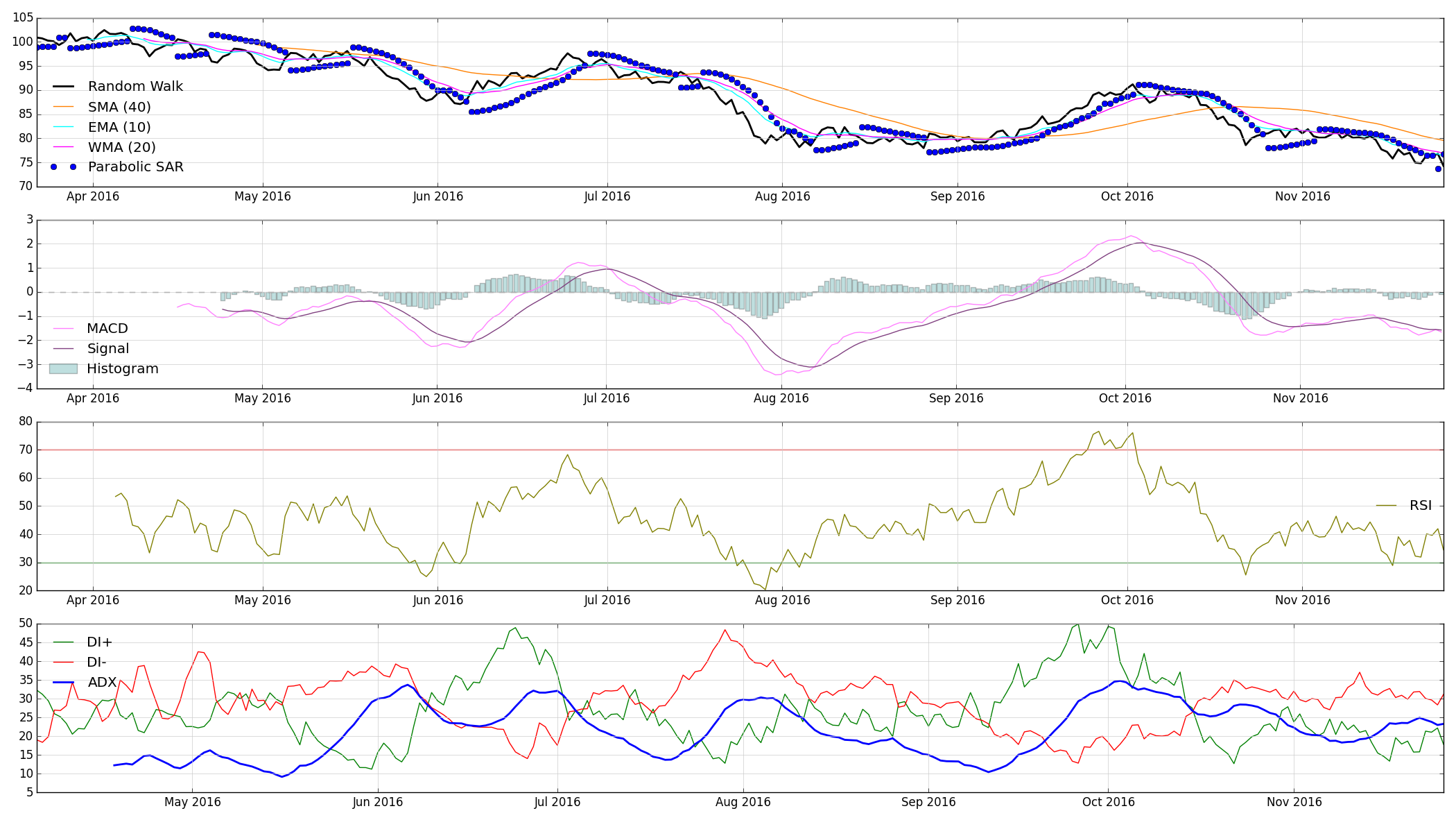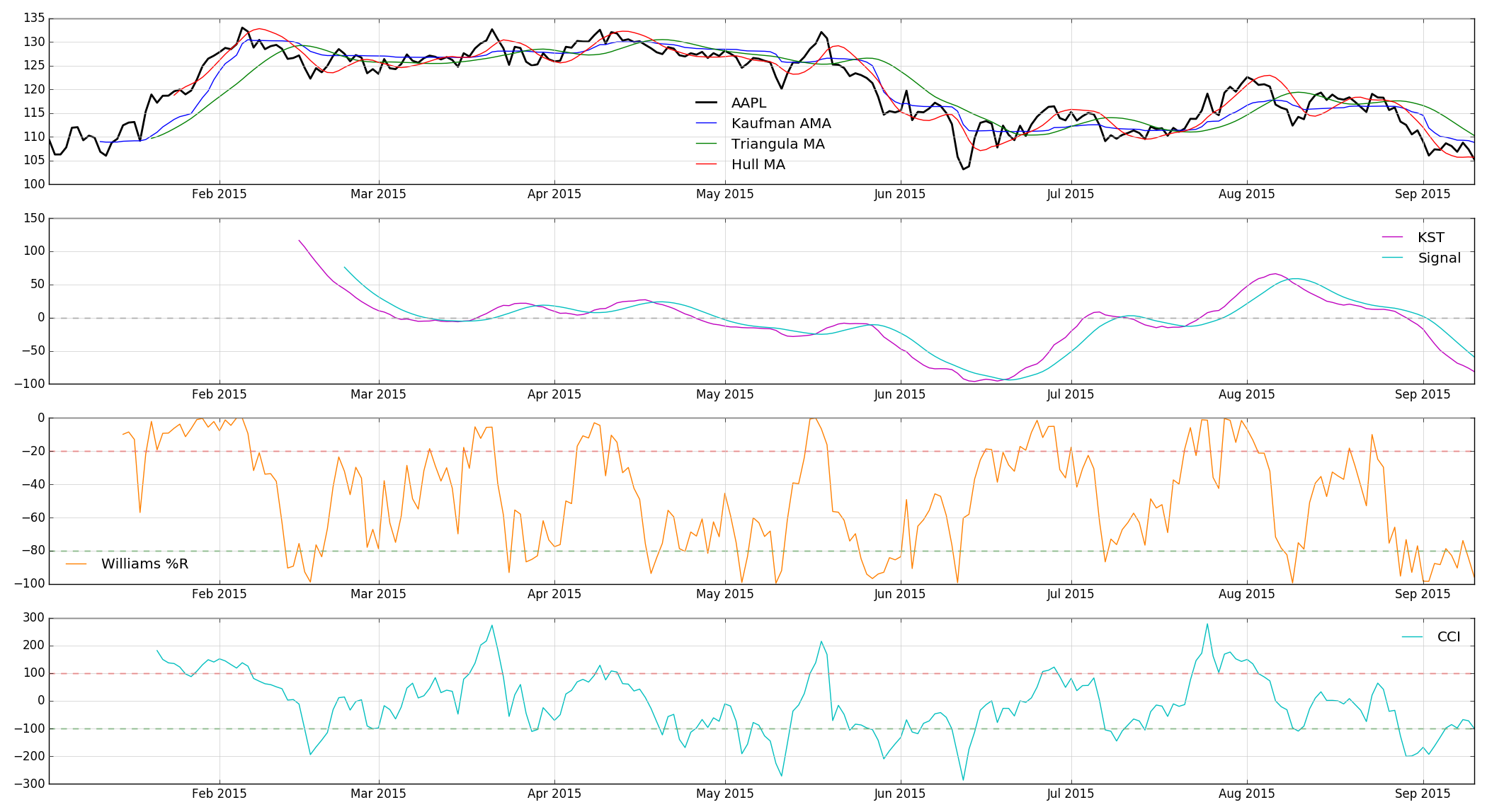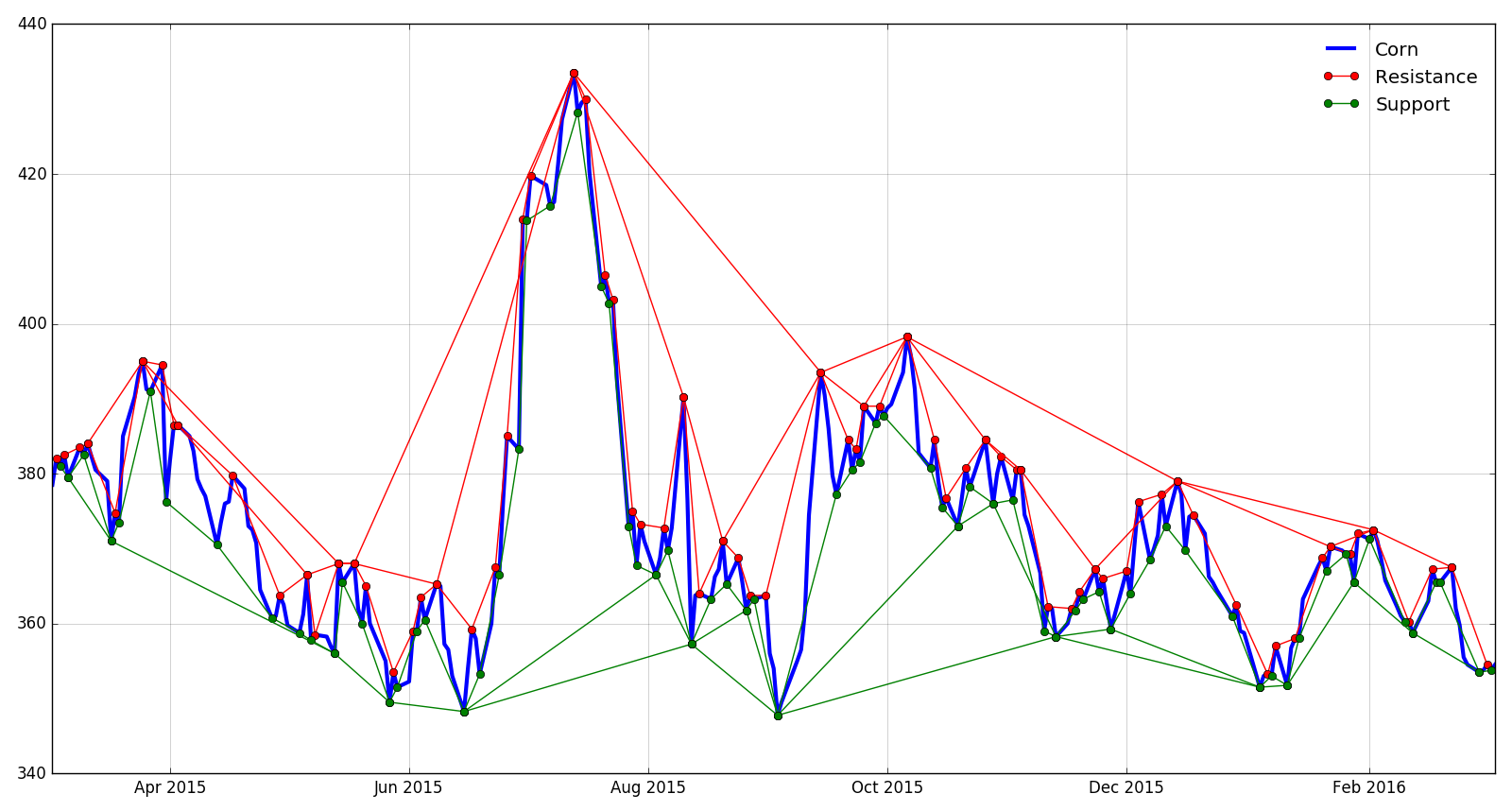# Indicators

70

3

19

6

### contributors# Indicators

Indicators is a Julia package offering efficient implementations of many technical analysis indicators and algorithms. This work is inspired by the TTR package in R and the Python implementation of TA-Lib, and the ultimate goal is to implement all of the functionality of these offerings (and more) in Julia. This package has been written to be able to interface with both native Julia `Array` types, as well as the `TS` time series type from the Temporal package. Contributions are of course always welcome for wrapping any of these functions in methods for other types and/or packages out there, as are suggestions for other indicators to add to the lists below.

## Implemented

### Moving Averages

• SMA (simple moving average)
• WMA (weighted moving average)
• EMA (exponential moving average)
• TRIMA (triangular moving average)
• KAMA (Kaufman adaptive moving average)
• MAMA (MESA adaptive moving average, developed by John Ehlers)
• HMA (Hull moving average)
• ALMA (Arnaud-Legoux moving average)
• SWMA (sine-weighted moving average)
• DEMA (double exponential moving average)
• TEMA (triple exponential moving average)
• ZLEMA (zero-lag exponential moving average)
• MMA (modified moving average)
• MLR (moving linear regression)
• Prediction
• Slope
• Intercept
• Standard error
• Upper & lower bound
• R-squared

### Momentum Indicators

• Momentum (n-day price change)
• ROC (rate of change)
• MACD (moving average convergence-divergence)
• RSI (relative strength index)
• ADX (average directional index)
• Parabolic SAR (stop and reverse)
• Fast & slow stochastics
• SMI (stochastic momentum indicator)
• KST (Know Sure Thing)
• Williams %R
• CCI (commodity channel index)
• Donchian channel
• Aroon indicator + oscillator

### Volatility Indicators

• Bollinger Bands
• Average True Range
• Keltner Bands

### Other

• Rolling/running mean
• Rolling/running standard deviation
• Rolling/running variance
• Rolling/running covariance
• Rolling/running correlation
• Rolling/running maximum
• Rolling/running minimum
• Rolling/running MAD (mean absolute deviation)
• Rolling/running quantiles

## Todo

• ~~Moving Linear Regression~~
• ~~KAMA (Kaufman adaptive moving average)~~
• ~~DEMA (double exponential moving average)~~
• ~~TEMA (tripe exponential moving average)~~
• ~~ALMA (Arnaud Legoux moving average)~~
• ~~Parabolic SAR~~
• ~~Williams %R~~
• ~~KST (know sure thing)~~
• ~~CCI (commodity channel index)~~
• ~~ROC (rate of change)~~
• ~~Momentum~~
• ~~Donchian Channel~~
• ~~Aroon Indicator / Aroon Oscillator~~
• ~~Stochastics~~
• ~~Slow Stochastics~~
• ~~Fast Stochastics~~
• ~~Stochastic Momentum Index~~
• ~~MMA (modified moving average)~~
• ~~ZLEMA (zero lag exponential moving average)~~
• Hamming moving average
• VWMA (volume-weighted moving average)
• VWAP (volume-weighted average price)
• EVWMA (elastic, volume-weighted moving average)
• VMA (variable-length moving average)
• Chaikin Money Flow
• Ultimate Oscillator
• OBV (on-balance volume)
• Too many more to name...always happy to hear suggestions though!

# Examples

#### Randomly generated data:#### Apple (AAPL) daily data from 2015:#### Corn futures daily data03/15/2016

27 days ago

193 commits